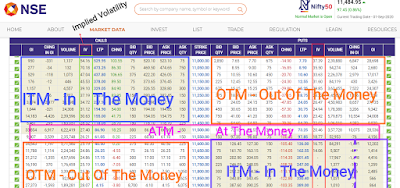IV in Option Premiums, It is Dependent Majorly on 3 Factors, However due to historically high levels of VIX and too much volatility in the market

# Implied Volatility IV in Option Premiums

Understand the nature of IV in option premiums and their relation with demand from the buyers. Generally higher IV corresponds to higher demand from option buyers but these days we see that IV is higher on PUT side even when there is bullishness indicated by price movements and OI data. Kindly help me in finding answers to this situation.

## Implied Volatility IV

As we know that,
Option premium = Intrinsic Value + Extrinsic Value
For Out Of The Money OTM,
Options Intrinsic Value = 0 (Always)
For IN The Money  ITM,
Options Intrinsic Value =|Spot - Strike Price|
Extrinsic value is applicable on both OTM and ITM strikes.

### 1.Risk Free Interest Rate Value

These two factors have time value associated with them. Risk Free Interest Value is simple. It is simply the interest that an option seller demands for time frame in which intends to keep his position open. So it would be more for an option that has more days left to expiry.

### 2.Risk Value

The second factor “Risk Value” is the most important component of an option premium. More the risk an option seller sees more premium he will demand.

### 3.Demand Value

The third factor takes into account the demand from the buyer and is simply a function of demand and supply. More the demand more is the price.

So it’s pretty complicated. So if we want to know that whether Extrinsic Value is more ,then the only factor that helps us is Implied Volatility.

Let us Discount Risk Free Interest Value at the moment as it is self explanatory.
I’ll simply represent it as following for sake of convenience.

IV = F(Risk Value seen by Sellers) + F(Demand from Buyers)

If IV is high it means that Extrinsic Value is High. So what can it be due to
1. More Risk Value that Option seller sees in writing the option
If IV is low it means that Extrinsic Value is low. So what can it be due to
1. Very less Risk Value that Option seller sees in writing the option

We see difference in IV on Call and Put side near Max Pain area on option chain. So as a buyer of an option if IV is high there may be a chance that Buyers are inclined more towards that particular side. This has to be validated by OI data that actually represent the true picture.

These days there have been instances where the OI data reflects Bullishness but IV is higher on PUT side. This simply means that PUT writers see very large risk in writing PUT options as VIX is very high and due to high volatility the bullishness may change back to bearishness anytime.

Relatively they see less risk in writing CALL option. Please lay emphasis on relatively word. Also the demand is high from buyers but overall the risk portion is too high for PUT that accounts for higher IV on PUT side despite buyers on CALL side.

Mathematically, I represent it as follows

IV (CALL) = RISK (CALL) + DEMAND (CALL)
IV (PUT ) = RISK (PUT) + DEMAND (PUT)

In Bullishness represented by OI data clearly DEMAND ( CALL ) > DEMAND ( PUT ).

Let us say, DEMAND ( CALL ) = 12
DEMAND ( PUT ) = 5

However due to historically high levels of VIX and too much volatility in the market.

RISK( PUT )>>>RISK( CALL )
Let us say, RISK ( PUT ) = 60
RISK ( CALL ) = 40

So overall IV( PUT )>IV( CALL )
Because IV( PUT )=5+60=65
IV( CALL ) = 12 + 40 = 52

This is over simplification bust just a way of understanding.
However in normal circumstances we may not see extraordinary RISK values and greater IV may correspond to greater demand by the buyers only.BLOGGER
Name

ltr
item
Markets Story: Understand The Nature of Implied Volatility (IV) in Option Premiums
Understand The Nature of Implied Volatility (IV) in Option Premiums
IV in Option Premiums, It is Dependent Majorly on 3 Factors, However due to historically high levels of VIX and too much volatility in the market